Past Year Questions: Structural Fasteners

# Past Year Questions: Structural Fasteners - Notes | Study Topic wise GATE Past Year Papers for Civil Engineering - Civil Engineering (CE)

 1 Crore+ students have signed up on EduRev. Have you?

Q.1 A 16 mm thick gusset plate is connected to the 12 mm thick flange plate of an l-section using fillet welds on both sides as shown in figure (not drawn to scale). The gusset plate is subjected to point of 350 kN acting at a distance of 100 mm from the flange plate. Size of fillet weld is 10 mm.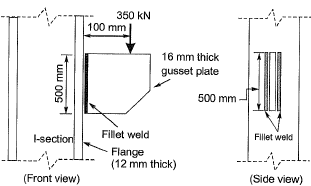The maximum resultant stress (in MPa, round off to 1 decimal place) on the fillet weld along the vertical plane would be_______.    [2019 : 2 Marks, Set-I]
Solution: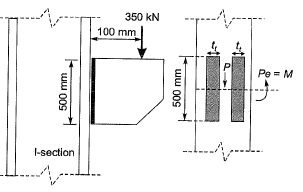P = 150 kN, e = 100 mm
tt = Effective throat thickness
tt = 0.7S
S = Size of weld = 10 mm
∴ tt = 0.7 x 10 = 7 mm
Shear stress due to direct force,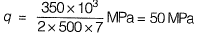Normal stress due to bending,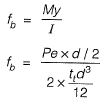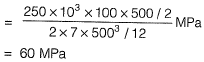Resultant stress,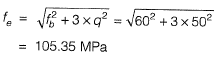Q.2 Four bolts P, Q, R and S of equal diameter are used for a bracket subjected to a load of 130 kN as shown in the figure.The force in bolt P is    [2018 : 2 Marks, Set-II]
(a) 32.50 kN
(b) 69.32 kN
(c) 82.50 kN
(d) 119.32 kN
Ans.
(B)
Solution: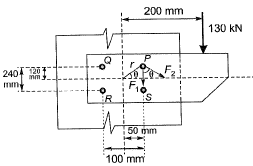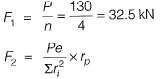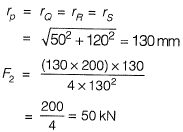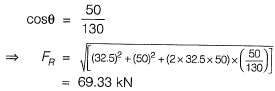Q.3  A fillet weld is simultaneously subjected to factored normal and shear stresses of 120 MPa and 50 MPa, respectively. As per IS 800 : 2007, the equivalent stress (in MPa. up to two decimal places) is _____.    [2018 : 1 Mark, Set-II]
Solution:

Factored normal stress, fa = 120 MPa
Factored shear stress, q = 50 MPa
According to IS-800 : 2007, clause 10.5.10.1.1 The equivalent stress,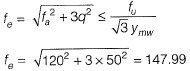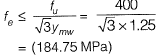So, equivalent stress 147.99 MPa.

Q.4  In a fillet weld, the direct shear stress and bending tensile stress are 50 MPa and 150 MPa, respectively. As per IS 800: 2007, the equivalent stress (in MPa, up to two decimal places) will be _____.    [2018 : 1 Mark, Set-I)
Solution:

Direct bending tensile stress,
fa = 150 MPa
Direct shear stress,
q = 50 MPa
According to IS 800: 2007, clause 10.5.10.1.1
The equivalent stress,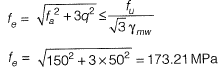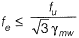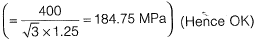So, equivalent stress of 173.21 MPa.

Q.5 Two plates of 8 mm thickness each are connected by a fillet weld of 6 mm thickness as shown in the figure.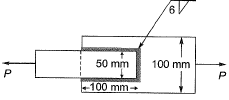The permissible stresses in the plate and the weld are 150 MPa and 110 MPa, respectively. Assuming the length of the weld shown in the figure to be the effective length, the permissible load P (in kN) is ____________ .    [2017 : 2 Marks, Set-II]
Solution: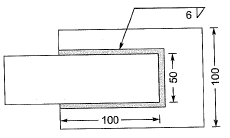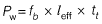= 110 (100 + 100 + 50) x 0.7 x 6
= 115.5 kN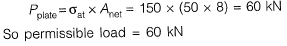Q.6 A column is subjected to a load through a bracket as shown in the figure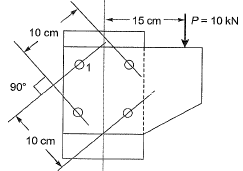The resultant force (in kN, up to one decimal place) in the bolt 1 is _________ .    [2017 : 2 Marks, Set-I]
Solution: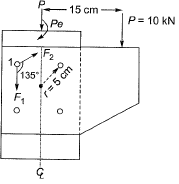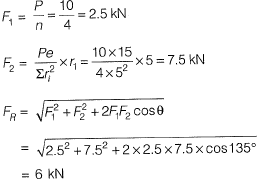Q.7 Two bolted plates under tension with alternative arrangement of bolt holes are shown in figures 1 and 2. The hole diameter, pitch, and gauge length are d, p and g, respectively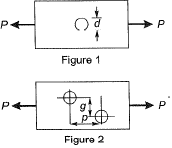Which one of the following conditions must be ensured to have higher net tensile capacity of configuration shown in figure 2 than that shown in figure 1?    [2016 : 2 Marks, Set-Il]
(a) p2 > 2gd
(b) p2 <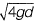(c) p2 > 4gd
(d) p > 4gd
Ans.
(C)
Solution: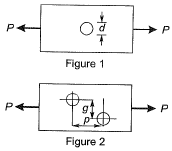Tensile strength of plate in arrangement (2) will be greater than in arrangement (1),
* As per IS code 800:2007 close 6.3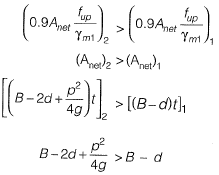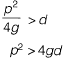Q.8 Two plates are connected by fillet welds of size 10 mm and subjected to tension, as shown in the figure. The thickness of each plate is 12 mm. The yield stress and the ultimate tensile stress of steel are 250 MPa and 410 MPa, respectively. The welding is done in the workshop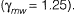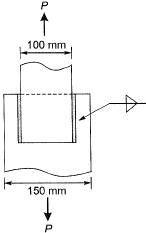As per the Limit State Method of IS 800 : 2007, the minimum length (rounded off to the nearest higher multiple of 5 mm) of each weld to transmit a force P equal to 270 kN (factored) is    [2016 : 2 Marks, Set-T]
(a) 90 mm
(b) 105 mm
(c) 110 mm
(d) 115 mm
Ans.
(B)
Solution:
Maximum force carried by plates,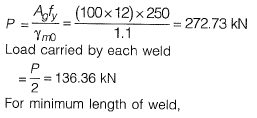Strength of weld = Load carried by weld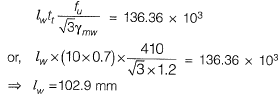= 105 mm (rounded off to nearest higher multiple of 5 mm)

Q.9 A bracket plate connected to a column flange transmits a load of 100 kN as shown in the following figure. The maximum force for which the bolts should be designed i s ________ kN.    [2015 : 2 Marks, Set-I]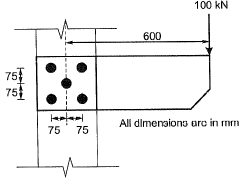Solution: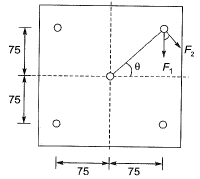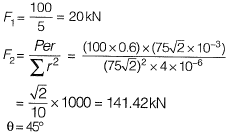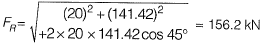Q.10 The tension and shear force (both in kN) in each bolt of the joint, as shown below, respectively are    [2014 : 2 Marks, Set-I]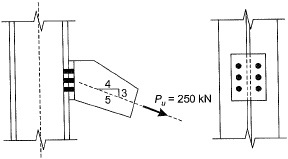(a) 30.33 and 20.00
(b) 30.33 and 25.00
(c) 33.33 and 20.00
(d) 33.33 and 25.00
Ans.
(D)
Solution: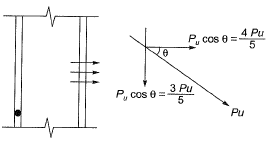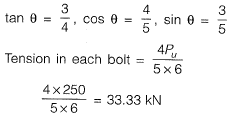Shear in each bolt =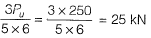Q.11 Two plates are connected by fillet welds of size 10 mm and subjected to tension, as shown in the sketch. The thickness of each plate is 12 mm. The yield stress and the ultimate tensile stress of steel are 250 MP and 410 MPa respectively. The welding is done in the workshop (λmm = 1.25). As per the Limit State Method of IS 800:2007, the minimum length (rounded off to the nearest higher multiple of 5 mm) of each weld to transmit a force P equal to   [2012 : 2 Marks]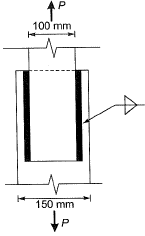(a) 100 mm
(b) 105 mm
(c) 110 mm
(d) 115 mm
Ans.
(B)
Solution: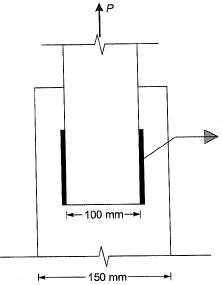Maximum force transmitted by plate = P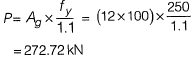Force resisted by weld,
P= 272.72 kN ...(i)
Let length of weld on either side = l
Size of weld (S) = 10 mm
force resisted by weld =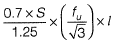Size= 10 mm
Throat thickness = 0.7 x 10 = 7 mm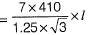...(ii)
From equations (i) and (ii),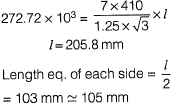Q.12 In a steel plate with bolted connection, the rupture of the net section is a mode of failure under    [2012 : 1 Mark]
(a) tension
(b) compression
(c) flexure
(d) shear
Ans.
(A)
Solution: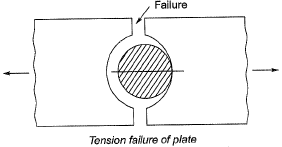Q.13 For the fillet weld of size ‘s’ shown in the adjoining figure the effective throat thickness is    [2011 : 1 Mark]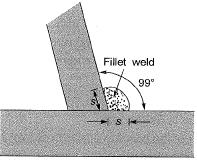(a) 0.61s
(b) 0.65s
(c) 0.70s
(d) 0.75s
Ans.
(B)
Solution: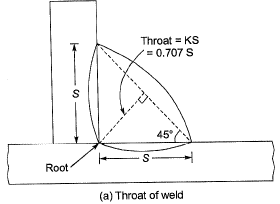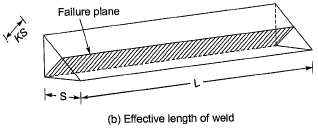Effective throat thickness is the shortest distance from the root of fillet weld to the face of the line joining the toes. The effective throat thickness should not be less than 3 mm.
Effective throat thickness = Ks.
where s is the size of weld in mm and K is a constant. The value of K depends upon the angle between the fusion faces.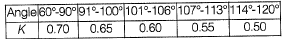Q.14 wo plates, subjected to direct tension, each of 10 mm thickness and having widths of 100 mm and 175 mm, respectively are to be fillet welded with an overlap of 200 mm. Given that the permissible weld stress is 110 MPa and the permissible stress in steel is 150 MPa, the length of the weld required using the maximum permissible weld size as per IS 800: 1984 is    [2010 : 2 Marks]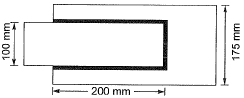(a) 245. 3 mm
(b) 229.2 mm
(c) 205.5 mm
(d) 194.8 mm
Ans.
(B)
Solution:
The maximum size of a fillet weld is obtained by subtracting 1.5 mm from the thickness of the thinner member to be jointed.
∴ S = 10 - 1.5 = 8.5 mm
Strength of the thinner plate
= 100 x 10 x 150 x 10-3 = 150 kN
Strength of the weld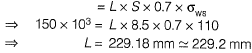Q.15  A double cover butt riveted joint is used to connect two flat plates of 200 mm width and 14 mm thickness as shown in the figure. There are twelve power driven rivets of 20 mm diameter at a pitch of 50 mm in both directions on either side of the plate. Two cover plates of 10 mm thickness are used. The capacity of the joint in tension considering bearing and shear ONLY, with permissible bearing and shear stresses as 300 MPa and 100 MPa respectively is    [2010 : 2 Marks]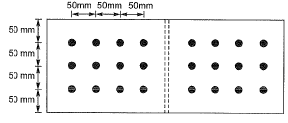(a) 1083.6 kN
(b) 871.32 kN
(c) 541.8 kN
(d) 433.7 kN
Ans.
(B)
Solution:
Strength of one rivet in double shear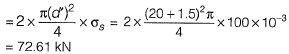Strength of the riveted joint in double shear
= 12 x 72.61 = 871.32 kN
Strength of one rivet in bearing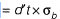= (20 + 1.5) x 14 x 300 x 10-3 = 90.3 kN
Strength of the riveted joint in bearing
= 12 x 90.3 = 1083.6 kN
Thus the strength of joint will be governed by shearing and it will be equal to 871.32 kN

The document Past Year Questions: Structural Fasteners - Notes | Study Topic wise GATE Past Year Papers for Civil Engineering - Civil Engineering (CE) is a part of the Civil Engineering (CE) Course Topic wise GATE Past Year Papers for Civil Engineering.
All you need of Civil Engineering (CE) at this link: Civil Engineering (CE)

## Topic wise GATE Past Year Papers for Civil Engineering

67 docs
 Use Code STAYHOME200 and get INR 200 additional OFF

## Topic wise GATE Past Year Papers for Civil Engineering

67 docs

Track your progress, build streaks, highlight & save important lessons and more!

,

,

,

,

,

,

,

,

,

,

,

,

,

,

,

,

,

,

,

,

,

;